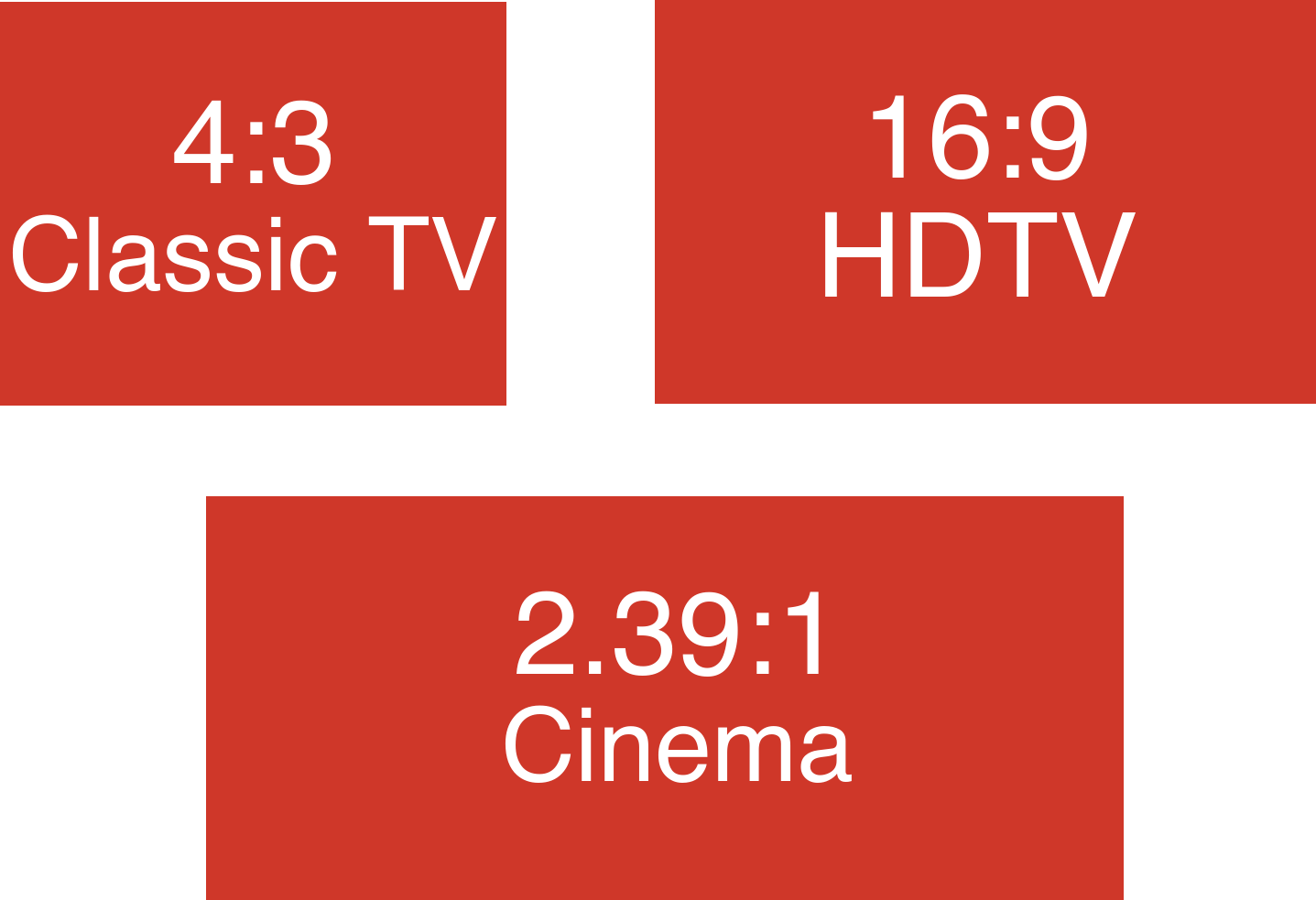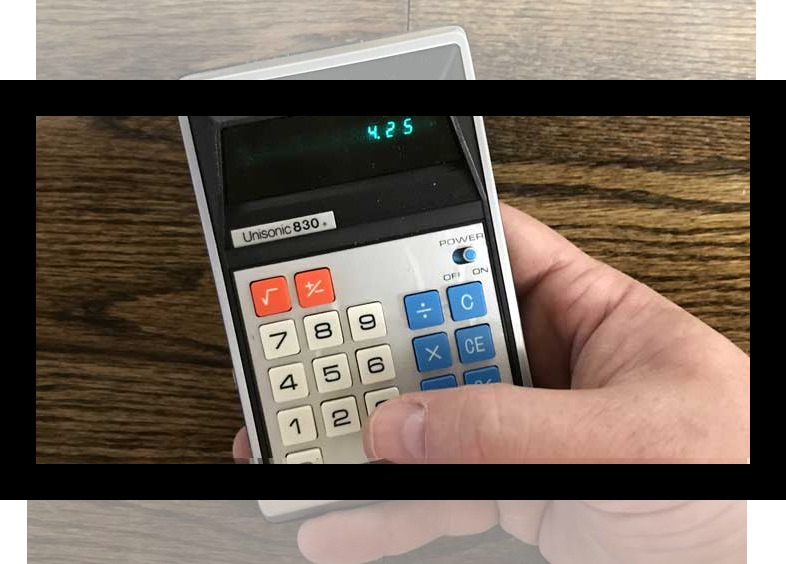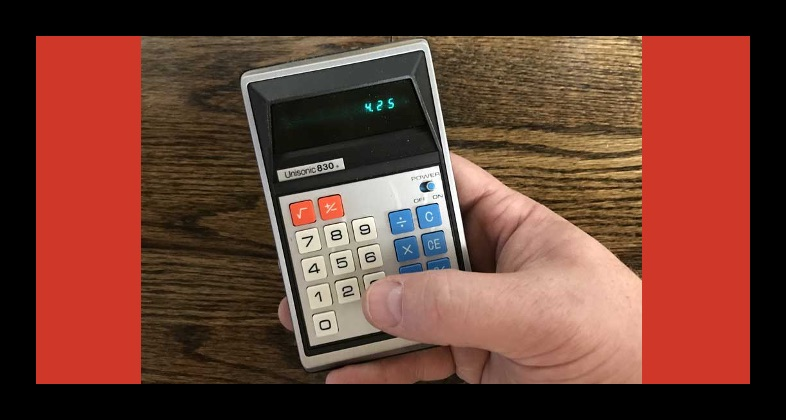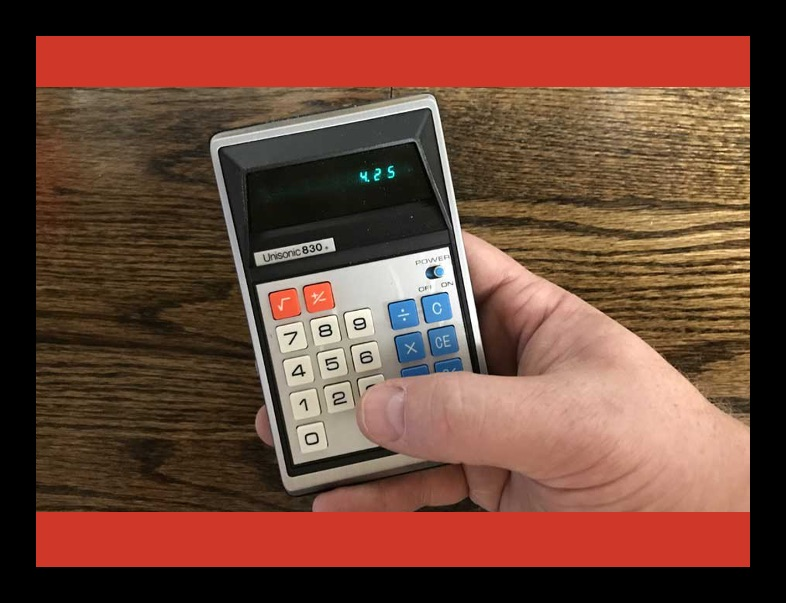# Aspect Ratio Calculator

The ultimate aspect ratio calculator calculates the aspect ratio for video or image sizes, scales a resolution using the same aspect ratio, compares resolutions, resizes images and video, and converts aspect ratios.

Enter any three aspect ratio values to calculate the fourth
Aspect Ratio One
:
=
Aspect Ratio Two
:
Reduce an aspect ratio to the simplest form
Aspect Ratio
:
Enter two aspect ratios to compare
Aspect Ratio One
:
Aspect Ratio Two
:
Scale an aspect ratio larger or smaller
Aspect Ratio
:

## Results:

Learn how we calculated this below

## How to Calculate an Aspect Ratio

An aspect ratio indicates the shape of a video screen or image. Screens display images as a grid of colored squares or rectangles in a grid, and the width and height of that grid is the resolution.

These squares or rectangles are called pixels. The aspect ratio is the ratio of the number of columns to the number of rows of pixels that make up a video display or image.

An aspect ratio is often expressed as reduced to a common format based on industry standards.

To find the video aspect ratio reduce the aspect ratio to either 16:9, 16:10, or 4:3. Some video aspect ratios cannot be evenly reduced into these ratios, in which case they should be reduced to the number of columns to 1 row of pixels.

Cinema projection screens often use a ratio of 2.35:1 or 2.4:1, for example. Learn more about these on our projector calculator.

### How to Reduce an Aspect Ratio

To reduce a ratio, find the greatest common factor of both the number of columns and the number of rows. Then divide the number of rows and the number of columns by the greatest common factor.

Example: let’s find the aspect ratio for a 3840 × 2160 resolution display.

The greatest common factor of both 3840 and 2160 is 240

num columns = 3840 ÷ 240 = 16
num rows = 2160 ÷ 240 = 9
aspect ratio = 16:9

### How to Scale an Aspect Ratio

Sometimes you need to scale an aspect ratio to a larger or smaller resolution. To scale an aspect ratio, multiply both sides of the resolution by the scale factor.

You can use our scale calculator to find the scale factor or scale measurements.

Example: let’s double the 4k 3840 and 2160 resolution to find the 8k resolution.

Multiply both parts of the resolution by 2

num columns = 3840 × 2 = 7680
num columns = 2160 × 2 = 4320

Thus, 4k scaled to 8k resolution is equal to 7680 x 4320.

## Common Image Aspect Ratios

While images come in many shapes and sizes, there are some aspect ratios that are more commonly used for images and photography.

Common aspect ratios for images and photography
Aspect Ratio Image Format
1:1 Square
3:4 Portrait
2:3 Portrait
5:7 Portrait
8:10 Portrait
4:3 Landscape
3:2 Landscape
7:5 Landscape
10:8 Landscape
16:9 Landscape

## Common Video Aspect Ratios

There are many aspect ratios used for various purposes in film and video, and some are more common than others. The most common is 16:9; see the table below for the most common ratios for each type of use.

Common aspect ratios for different video uses
Video Format Common Aspect Ratios
Cinema 1.85:1, 2.39:1
TV Video 4:3, 16:9
Computers 4:3, 16:10

See a full list modern aspect ratios and resolutions.## How to Convert Video to a Different Aspect Ratio

Sometimes video in one aspect ratio needs to be displayed on a screen that is a different resolution, for example, when displaying standard definition 4:3 TV on a high definition 16:9 display.

There are a few different ways to display video that is not the same aspect ratio as the screen.

### Display a Different Aspect Ratio by Cropping

The video can be zoomed so that there is no extra space, which will crop part of the video.

For example, a 4:3 video can be zoomed to scale the width to that of a 16:9 screen, cropping the top and bottom of the video.Cropping the video results in losing the top and bottom of the image

### Display a Different Aspect Ratio by Pillar-boxing

The video can be pillar-boxed, keeping the video’s height the same as the display when the video aspect ratio is thinner than the display aspect ratio.

For example, a 4:3 video can be zoomed, scaling the video’s height to the height of the display, resulting in bars on either side of the video.Pillar-boxing the video results in black bars on the left and right of the image

### Display a Different Aspect Ratio by Letterboxing

The video can be letterboxed, keeping the video’s width the same as the display when the video aspect ratio is wider than the display aspect ratio.

For example, a 16:9 video can be zoomed so that the video’s width is the same as the display’s width, resulting in bars on the top and bottom of the video.Letterboxing the video results in black bars on the top and bottom of the image

You might be interested in our TV viewing distance calculator to calculate the resolution and size of TV you should get based on your seating distance.